Courses

# One Dimensional Unsteady Conduction & Free & Forced Convection - MCQ Test 2

## 25 Questions MCQ Test GATE Mechanical (ME) 2022 Mock Test Series | One Dimensional Unsteady Conduction & Free & Forced Convection - MCQ Test 2

Description
This mock test of One Dimensional Unsteady Conduction & Free & Forced Convection - MCQ Test 2 for Mechanical Engineering helps you for every Mechanical Engineering entrance exam. This contains 25 Multiple Choice Questions for Mechanical Engineering One Dimensional Unsteady Conduction & Free & Forced Convection - MCQ Test 2 (mcq) to study with solutions a complete question bank. The solved questions answers in this One Dimensional Unsteady Conduction & Free & Forced Convection - MCQ Test 2 quiz give you a good mix of easy questions and tough questions. Mechanical Engineering students definitely take this One Dimensional Unsteady Conduction & Free & Forced Convection - MCQ Test 2 exercise for a better result in the exam. You can find other One Dimensional Unsteady Conduction & Free & Forced Convection - MCQ Test 2 extra questions, long questions & short questions for Mechanical Engineering on EduRev as well by searching above.
QUESTION: 1

### Which one of the following statements is correct?   The curve for unsteady state cooling or heating of bodies

Solution:

Ans. (b)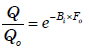QUESTION: 2

Solution:

Ans. (d)

QUESTION: 3

### The properties of mercury at 300 K are: density = 13529 kg/m3, specific heat at constant pressure = 0.1393 kJ/kg-K, dynamic viscosity = 0.1523 ×10-2 N.s/m2 and thermal conductivity = 8.540 W/mK. The Prandtl numberof the mercury at 300 K is:

Solution:

Ans. (a)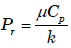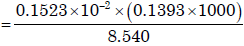= 0.0248

QUESTION: 4

Free convection flow depends on all of the following EXCEPT  [

Solution:

Ans. (d)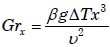QUESTION: 5

A cube at high temperature is immersed in a constant temperature bath. It loses heat from its top, bottom and side surfaces with heat transfer coefficient of h1, h2 and h3 respectively. The average heat transfer coefficient for the cube is:

Solution:

Ans. (d)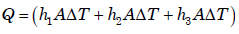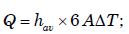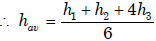QUESTION: 6

(a)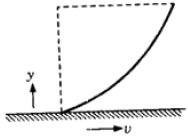(b)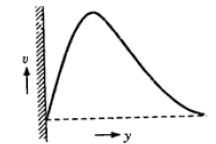(c)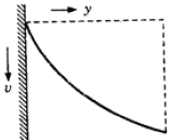(d)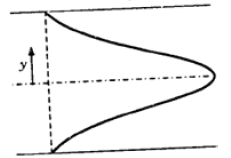Match the velocity profiles labelled A, B, C and D with the following situations:
1. Natural convection
2. Condensation
3. Forced convection
4. Bulk viscosity ≠ wall viscosity
5. Flow in pipe entrance

Select the correct answer using the codes given below:
Codes: A B C D                A B C D
(a) 3 2 1 5             (b) 1 4 2 3
(c) 3 2 1 4             (d) 2 1 5 3

Solution:

Ans. (a) It provides right matching

QUESTION: 7

Match List-I (Dimensionless quantity) with List-II (Application) andselect the correct answer using the codes given below the lists: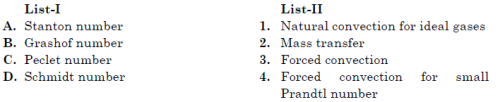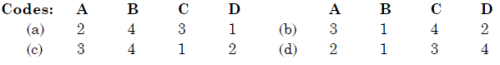Solution:

Ans. (b) The correct matching for various dimensionless quantities is provided by
code (b)

QUESTION: 8

When there is a flow of fluid over a flat plate of length 'L', the average heat transfer coefficient is given by (Nux = Local Nusselt number; other symbols have the usual meaning)

Solution:

Ans. (c)

QUESTION: 9

For steady, uniform flow through pipes with constant heat flux supplied to the wall, what is the value of Nusselt number?

Solution:

Ans. (a)

QUESTION: 10

For steady, uniform flow through pipes with constant heat fluxsupplied to the wall, what is the value of Nusselt number?

Solution:

Ans. (a)

QUESTION: 11

A fluid of thermal conductivity 1.0 W/m-K flows in fully developed flow with Reynolds number of 1500 through a pipe of diameter 10 cm. The heat transfer coefficient for uniform heat flux and uniform wall
temperature boundary conditions are, respectively.

Solution: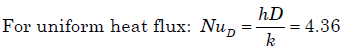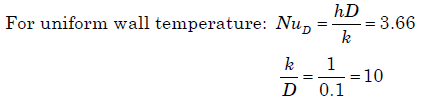QUESTION: 12

A 320 cm high vertical pipe at 150°C wall temperature is in a room withstill air at 10°C. This pipe supplies heat at the rate of 8 kW into theroom air by natural convection. Assuming laminar flow, the height ofthe pipe needed to supply 1 kW only is

Solution:

Ans. (b) For vertical pipe characteristic dimension is the length of the pipe.
For laminar flow Nu = (Gr.Pr)1/4

h become independent of length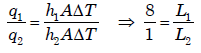L2 = 40cm

QUESTION: 13

The average Nusselt number in laminar natural convection from avertical wall at 180°C with still air at 20°C is found to be 48. If the wall temperature becomes 30°C, all other parameters remaining same, the average Nusselt number will be:

Solution:

Correct Answer :- c

Explanation : Nu2/Nu1 = Δt2/Δt1

= 60/160

Nu2 = 24

QUESTION: 14

Nusselt number for a pipe flow heat transfer coefficient is given by the equation NuD = 4.36. Which one of the following combinations ofconditions does exactly apply for use of this equation?

Solution:

Ans. (d)

QUESTION: 15

Nusselt number for fully developed turbulent flow in a pipe is given by  The values of a and b are:

Solution:

Ans. (c) Fully developed turbulent flow inside tubes (internal diameter D):
Dittus-Boelter Equation:
Nusselt number, NuD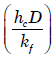= 0.023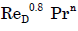where, n = 0.4 for heati=g (Tw > Tf) and n = 0.3 for cooling (Tw < Tf)

QUESTION: 16

Which one of the following numbers represents the ratio of kinematic viscosity to the thermal diffusivity?

Solution:

Ans. (b)

QUESTION: 17

In free convection heat transfer transition from laminar to turbulentflow is governed by the critical value of the

Solution:

Ans. (d)

QUESTION: 18

Which one of the following non-dimensional numbers is used fortransition from laminar to turbulent flow in free convection?

Solution:

Ans. (d) Laminar to Turbulent Transition: Just as for forced convection, a boundary layer will form for free convection.
The insulating film will be relatively thin toward the leading edge of the surface
resulting in a relatively high convection coefficient. At a Rayleigh number of about 10the flow over a flat plate will transition to a turbulent pattern. The increased
turbulence inside the boundary layer will enhance heat transfer leading to relative high convection coefficients, much like forced convection.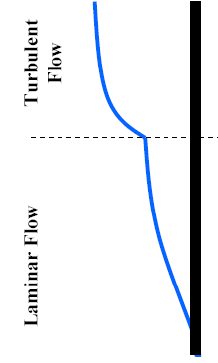Ra < 109Laminar flow [Vertical flat plate]
Ra > 109 Turbulent flow [Vertical flat plate]

QUESTION: 19

In free convection heat transfer transition from laminar to turbulent flow is governed by the critical value of the

Solution:

Ans. (d)

QUESTION: 20

Match List-I (Type of heat transfer) with List-II (Governing dimensionless parameter) and select the correct answer:
List-I                                                              List-II
A. Forced convection                                1. Reynolds, Grashof and Prandtl number
B. Natural convection                                2. Reynolds and Prandtl number
C. Combined free and forced convection  3. Fourier modulus and Biot number
D. Unsteady conduction with                     4. Prandtl number and Grash of convection at surface number
Codes: A B C D             A B C D
(a) 2 1 4 3         (b) 3 4 1 2
(c) 2 4 1 3         (d) 3 1 4 2

Solution:

Ans. (c)

QUESTION: 21

Match List-I (Process) with List-II (Predominant parameter associated with the process) and select the correct answer using the codes given below the lists:
List-I                                                     List-II
A. Mass transfer                          1. Reynolds Number
B. Forced convection                   2. Sherwood Number
C. Free convection                      3. Mach Number
D. Transient conduction              4. Biot Number
5. Grashoff Number
Codes: A B C D                            A B C D
(a) 5 1 2 3                         (b) 2 1 5 4
(c) 4 2 1 3                         (d) 2 3 5 4

Solution:

Ans. (b)

QUESTION: 22

Data are given below. Solve the problems and choose correct answers.
Heat is being transferred by convection from water at 48°C to a glass plate whose surface that is exposed to the water is at 40°C. The thermal conductivity of water is 0.6 W/mK and the thermal conductivity of
glass is 1.2 W/mK. The spatial Water gradient of temperature in the water at the water-glass interface is dT/dy =1 × 104 K/m.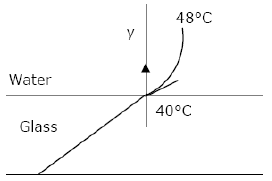Q. The value of the temperature gradient in the glass at the water-glass interface in k/m is:

Solution:

Ans. (c) Kw = 0.6W/mK; KG =1.2 W/mK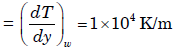The spatial gradient of temperature in water at the water-glass interface

At Water glass interface,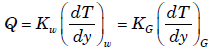or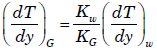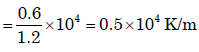QUESTION: 23

Data are given below. Solve the problems and choose correct answers.
Heat is being transferred by convection from water at 48°C to a glass plate whose surface that is exposed to the water is at 40°C. The thermal conductivity of water is 0.6 W/mK and the thermal conductivity of
glass is 1.2 W/mK. The spatial Water gradient of temperature in the water at the water-glass interface is dT/dy =1 × 104 K/m.Q. The heat transfer coefficient h in W/m2K is:

Solution:

Ans. (d) Heat transfer per unit area q = h ( Tf – Ti)

or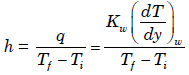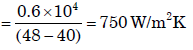QUESTION: 24

Match List-I (Phenomenon) with List-II (Associated dimensionless parameter) and select the correct answer using the code given below the lists: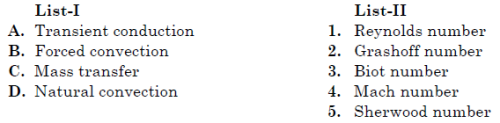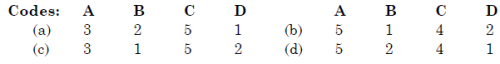Solution:

Ans. (c)

QUESTION: 25

For natural convective flow over a vertical flat plate as shown in the given figure, the governing differential
equation for momentum is: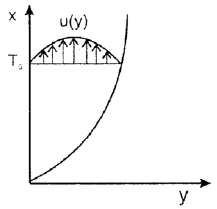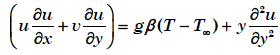If equation is non-dimensionalized by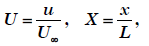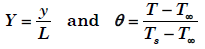then the term gβ (T -  T) , is equal to:

Solution:

Ans. (d)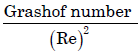gives dimensionless number which signifies whether flow is forced or free connection.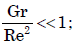Forced convection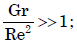Natural convection